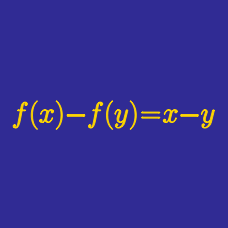Algebra

# Functional Equations - Substitution

Find all functions $f: \mathbb{R} \backslash \{ 0, 1 \} \rightarrow \mathbb{R}$ such that

$f \left( \frac{a}{a-1} \right) - 11 f \left( \frac{ a-1} {a} \right) = 0,$

for $a \neq 0, 1$.

A function $f(x)$ from the positive integers to the reals satisfies
1) $f(1) = 792$
2) $f(1) + f(2) + \ldots + f(n) = n^2 f(n)$ for any positive integer $n$.

What is the value of $f(11)$?

Consider the function $f(x)$ satisfying

$\left(x^2-x+1\right)f\left(x^2\right)=f(x), f(3)=3.$

If the value of $f(-9)$ can be expressed as $\frac{a}{b},$ where $a$ and $b$ are coprime positive integers, what is the value of $a+7b?$

A function $f: \mathbb{R} \backslash \{ 0, 1 \} \rightarrow \mathbb{R}$ satisfies

$f(x) + f \left( \frac{1}{1-x} \right) = 5 x,$

for $x \neq 0, 1$. What is the value of $40 f( 5)$?

Suppose that $f$ satisfies the following functional equation: $2f(x)+3f\left(\frac{3x+29}{x-3}\right)=100x+80.$ What is the value of $f(4)?$

×